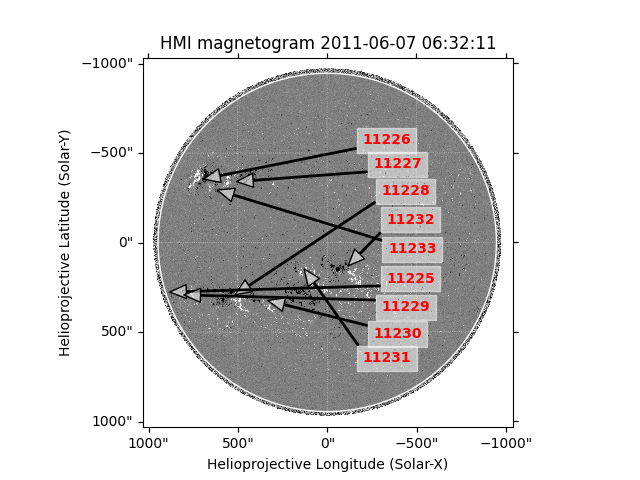# Overplotting SRS active region locations on a magnetograms#

How to find and plot the location of an active region on an HMI magnetogram.

import matplotlib.pyplot as plt
import numpy as np

import sunpy.coordinates
import sunpy.data.sample
import sunpy.map
from sunpy.io.special import srs


For this example, we will start with the sample data. We need an HMI file and use it to create a map, and the SRS table which contains a list of active regions. Both of these data can be downloaded with Fido.

smap = sunpy.map.Map(sunpy.data.sample.HMI_LOS_IMAGE)


We only need the rows which have ‘ID’ = ‘I’ or ‘IA’.

srs_table = srs_table[np.logical_or(srs_table['ID'] == 'I', srs_table['ID'] == 'IA')]


Now we extract the latitudes, longitudes and the region numbers.

lats = srs_table['Latitude']
lngs = srs_table['Longitude']
numbers = srs_table['Number']


Let’s plot the results by defining coordinates for each location.

fig = plt.figure()
# Passing vmin/vmax to plot does not work since
# a normalisation is set on the map. So we have to
# work around it like so:
smap.plot_settings["norm"].vmin = -150
smap.plot_settings["norm"].vmax = 150
smap.plot(axes=ax)
smap.draw_limb(axes=ax)

# Add a text box and arrow pointing to each active region
lat_text = -40
transparent_white = (1, 1, 1, 0.5)
for num, lng, lat in zip(numbers, lngs.value, lats.value):
ax.annotate(num, (lng, lat),
xytext=(320, lat_text),
xycoords=ax.get_transform('heliographic_stonyhurst'),
backgroundcolor=transparent_white,
color='red',
fontweight='bold',